Introduction

This article describes how the factorial and Gamma functions for non-integer arguments where implemented for the big-math library.

For an introduction into the Gamma function see Wikipedia: Gamma Function

Attempt to use Euler’s definition as an infinite product

Euler’s infinite product definition is easy to implement, but I have some doubts about its usefulness to calculate the result with the desired precision.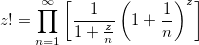public static BigDecimal factorialUsingEuler(BigDecimal x, int steps, MathContext mathContext) {
MathContext mc = new MathContext(mathContext.getPrecision() * 2, mathContext.getRoundingMode());

BigDecimal product = BigDecimal.ONE;
for (int n = 1; n < steps; n++) {
BigDecimal factor = BigDecimal.ONE.divide(BigDecimal.ONE.add(x.divide(BigDecimal.valueOf(n), mc), mc), mc).multiply(pow(BigDecimal.ONE.add(BigDecimal.ONE.divide(BigDecimal.valueOf(n), mc), mc), x, mc), mc);
product = product.multiply(factor, mc);
}

return product.round(mathContext);
}

Running with increasing number of steps shows that this approach will not work satisfactorily.

5! in       1 steps = 1
5! in      10 steps = 49.950049950049950050
5! in     100 steps = 108.73995188474609004
5! in    1000 steps = 118.80775820319167518
5! in   10000 steps = 119.88007795802040268
5! in  100000 steps = 119.98800077995800204
5! in 1000000 steps = 119.99880000779995800

Using Spouge’s Approximation

After reading through several pages of related material I finally find a promising approach: Spouge’s approximation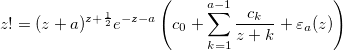where a is an arbitrary positive integer that can be used to control the precision and the coefficients are given by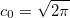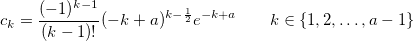Please note that the coefficients are constants that only depend on a and not on the input argument to factorial.

The relative error when omitting the epsilon part is bound to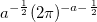It is nice to have a function that defines the error, normally I need to empirically determine the error for a sensible range of input arguments and precision.

Expected error of Spouge’s Approximation

Lets implement the error formula and see how it behaves.

public static BigDecimal errorOfFactorialUsingSpouge(int a, MathContext mc) {
return pow(BigDecimal.valueOf(a), BigDecimal.valueOf(-0.5), mc).multiply(pow(TWO.multiply(pi(mc), mc), BigDecimal.valueOf(-a-0.5), mc), mc);
}

Instead of plotting the error bounds directly, I determine the achievable precision using -log10(error).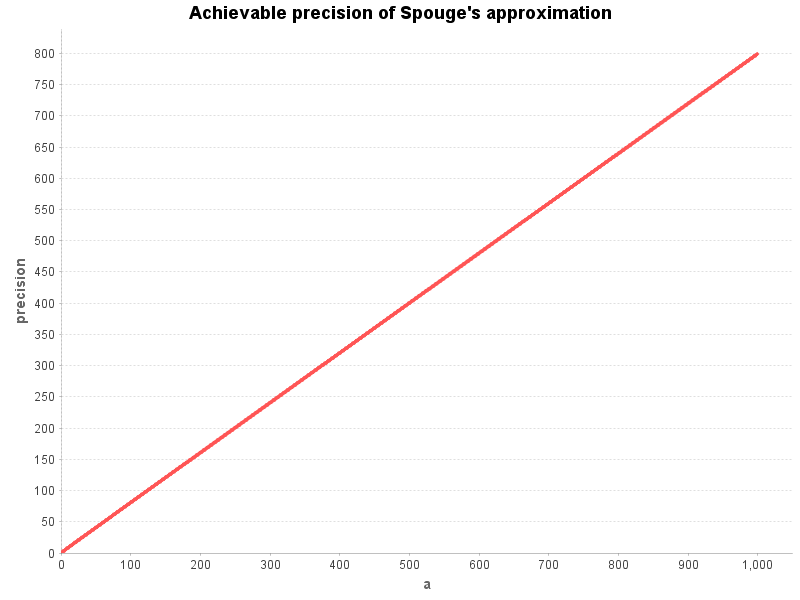Using the relative error formula of Spouge’s approximation we see that the expected precision is pretty linear to the chosen value of a for the values [1..1000] (which are a sensible range for the precision the users of the function will use).

This will make it easy to calculate a sensible value for a from the desired precision.

Note: While testing this I found a bug in log(new BigDecimal("6.8085176335035800378E-325")). Fixed it before it could run away.

Caching Spouge’s coefficients (depending on precision)

The coefficients depend only on the value of a.

We can cache the coefficients for every value of a that we need:

private static Map<Integer, List<BigDecimal>> spougeFactorialConstantsCache = new HashMap<>();

private static List<BigDecimal> getSpougeFactorialConstants(int a) {
return spougeFactorialConstantsCache.computeIfAbsent(a, key -> {
List<BigDecimal> constants = new ArrayList<>(a);
MathContext mc = new MathContext(a * 15/10);

BigDecimal c0 = sqrt(pi(mc).multiply(TWO, mc), mc);

boolean negative = false;
BigDecimal factor = c0;
for (int k = 1; k < a; k++) {
BigDecimal bigK = BigDecimal.valueOf(k);
BigDecimal ck = pow(BigDecimal.valueOf(a-k), bigK.subtract(BigDecimal.valueOf(0.5), mc), mc);
ck = ck.multiply(exp(BigDecimal.valueOf(a-k), mc), mc);
ck = ck.divide(factorial(k - 1), mc);
if (negative) {
ck = ck.negate();
}

negative = !negative;
}

return constants;
});
}

Calculating the coefficients becomes quite expensive with higher precision.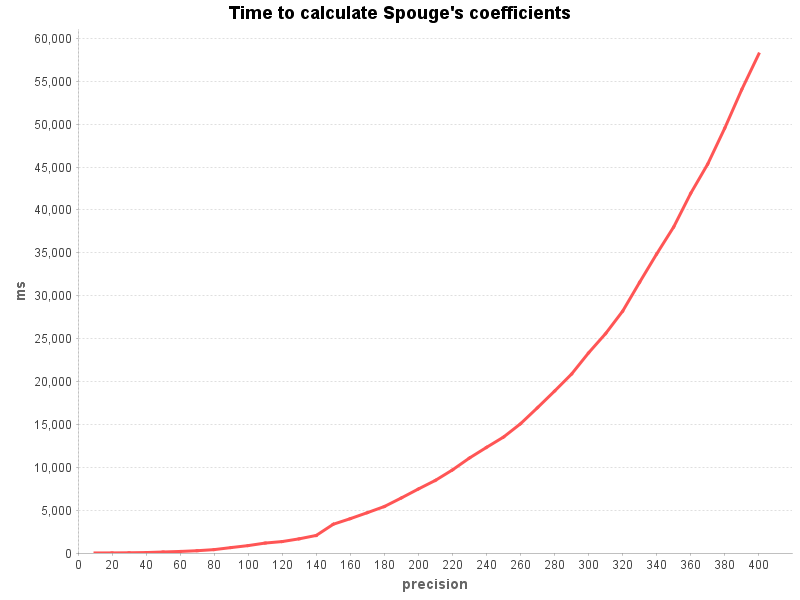This will need to be explained in the javadoc of the method.

Spouge’s approximation with pre-calculated constants

Now that we have the coefficients for a specific value of a we can implement the factorial method:

public static BigDecimal factorialUsingSpougeCached(BigDecimal x, MathContext mathContext) {
MathContext mc = new MathContext(mathContext.getPrecision() * 2, mathContext.getRoundingMode());

int a = mathContext.getPrecision() * 13 / 10;
List<BigDecimal> constants = getSpougeFactorialConstants(a);

BigDecimal bigA = BigDecimal.valueOf(a);

boolean negative = false;
BigDecimal factor = constants.get(0);
for (int k = 1; k < a; k++) {
BigDecimal bigK = BigDecimal.valueOf(k);
negative = !negative;
}

result = result.multiply(exp(x.negate().subtract(bigA, mc), mc), mc);
result = result.multiply(factor, mc);

return result.round(mathContext);
}

Let’s calculate first the factorial function with constant precision over a range of input values.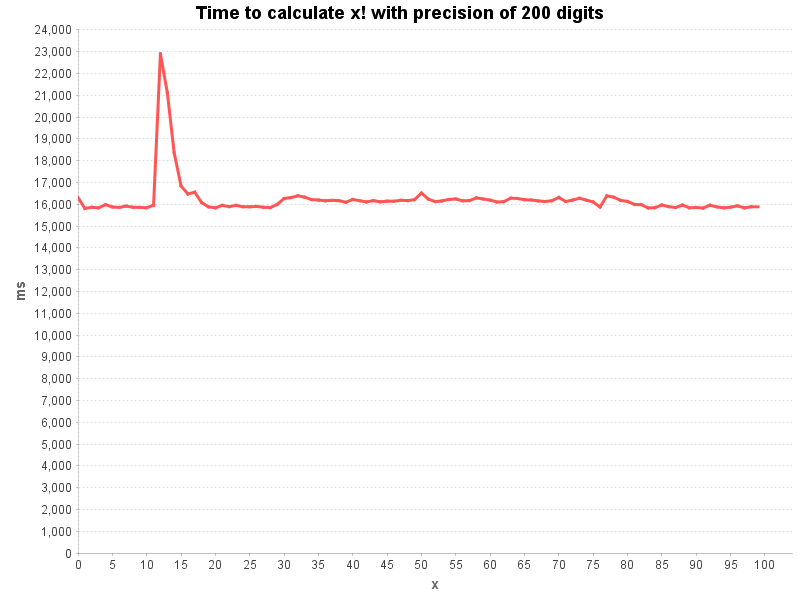Looks like the argument x does not have much influence on the calculation time.

More interesting is the influence that the precision has on the calculation time. The following chart was measured by calculating 5! over a range of precisions: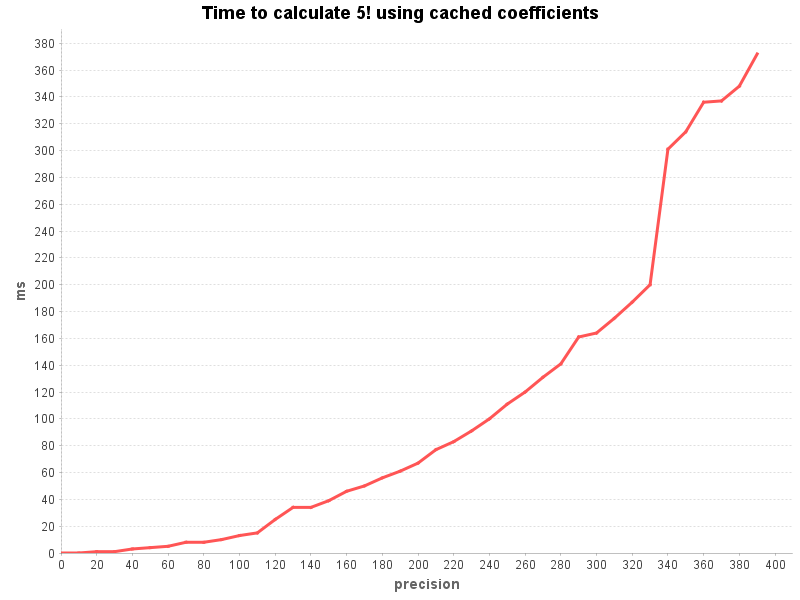Gamma function

The implementation of the Gamma function is trivial, now that we have a running factorial function.

public static BigDecimal gamma(BigDecimal x, MathContext mathContext) {
return factorialUsingSpougeCached(x.subtract(ONE), mathContext);
}

Polishing before adding it to BigDecimalMath

Before committing the new methods factorial() and gamma() to BigDecimalMath I need to do some polishing…

The access to the cache must be synchronized to avoid race conditions.

Most important is optimizing the calculation for the special cases of x being integer values which can be calculated much faster by calling BigDecimalMath.factorial(int).

Lots of unit tests of course! As usual Wolfram Alpha provides some nice reference values to prove that the calculations are correct (at least for the tested cases).

Writing javadoc takes also some time (and thoughts).

You can check out the final version in github: BigComplexMath.java

Release 2.0.0 of big-math library supports now complex numbers

The easter week-end was the perfect time to polish and release version 2.0.0 of the big-math library.

The class BigComplex represents complex numbers in the form (a + bi).
It follows the design of BigComplex with some convenience improvements like overloaded operator methods.

• re
• im
• subtract(BigComplex)
• subtract(BigComplex, MathContext)
• subtract(BigDecimal)
• subtract(BigDecimal, MathContext)
• subtract(double)
• multiply(BigComplex)
• multiply(BigComplex, MathContext)
• multiply(BigDecimal)
• multiply(BigDecimal, MathContext)
• multiply(double)
• divide(BigComplex)
• divide(BigComplex, MathContext)
• divide(BigDecimal)
• divide(BigDecimal, MathContext)
• divide(double)
• reciprocal(MathContext)
• conjugate()
• negate()
• abs(MathContext)
• angle(MathContext)
• absSquare(MathContext)
• isReal()
• re()
• im()
• round(MathContext)
• hashCode()
• equals(Object)
• strictEquals(Object)
• toString()
• valueOf(BigDecimal)
• valueOf(double)
• valueOf(BigDecimal, BigDecimal)
• valueOf(double, double)
• valueOfPolar(BigDecimal, BigDecimal, MathContext)
• valueOfPolar(double, double, MathContext)

A big difference to BigDecimal is that BigComplex.equals() implements the mathematical equality and not the strict technical equality.
This was a difficult decision because it means that BigComplex behaves slightly different than BigDecimal but considering that the strange equality of BigDecimal is a major source of bugs we decided it was worth the slight inconsistency.

If you need the strict equality use BigComplex.strictEquals().

The class BigComplexMath is the equivalent of BigDecimalMath and contains mathematical functions in the complex domain.

• sin(BigComplex, MathContext)
• cos(BigComplex, MathContext)
• tan(BigComplex, MathContext)
• asin(BigComplex, MathContext)
• acos(BigComplex, MathContext)
• atan(BigComplex, MathContext)
• acot(BigComplex, MathContext)
• exp(BigComplex, MathContext)
• log(BigComplex, MathContext)
• pow(BigComplex, long, MathContext)
• pow(BigComplex, BigDecimal, MathContext)
• pow(BigComplex, BigComplex, MathContext)
• sqrt(BigComplex, MathContext)
• root(BigComplex, BigDecimal, MathContext)
• root(BigComplex, BigComplex, MathContext)

Aurora Borealis (Northern Lights) in Tromsø

To celebrate my fiftieth birthday the whole family had a great vacation in Tromsø to see the northern lights.

The following videos where all taken using a wireless remote control with programmable interval.

The single shots where joined using ffmpeg.

#!/bin/sh
# $1 = framerate (for aurora timelapse use 1 to 4) #$2 = start number of first image
# $3 = output file (with .mp4 extension) ffmpeg -y -r "$1" -start_number "$2" -i IMG_%04d.JPG -s hd1080 -vf "framerate=fps=30:interp_start=0:interp_end=255:scene=100" -vcodec mpeg4 -q:v 1 "$3

In a few cases the images where a bit underexposed and needed to be brightened.
This was done with a simple shell script using the imagemagick convert.

#!/bin/sh

mkdir modulate150

for i in *.JPG
do
convert $i -modulate 150% modulate150/$i
done

This describes a way to improve the performance of a BigDecimal based implementation of Newton’s Method
by adapting the precision for every iteration to the maximum precision that is actually possible at this step.

As showcase I have picked the implementation of Newton’s Method to calculate the natural logarithm of a BigDecimal value with a determined precision.

The source code is available on github: big-math.

Here the mathematical formulation of the algorithm:

$$\require{AMSmath}$$
$$\displaystyle y_0 = \operatorname{Math.log}(x),$$
$$\displaystyle y_{i+1} = y_i + 2 \frac{x – e^{y_i} }{ x + e^{y_i}},$$
$$\displaystyle \ln{x} = \lim_{i \to \infty} y_i$$

Here a straightforward implementation:

private static final BigDecimal TWO = valueOf(2);

public static BigDecimal logUsingNewtonFixPrecision(BigDecimal x, MathContext mathContext) {
if (x.signum() <= 0) {
throw new ArithmeticException("Illegal log(x) for x <= 0: x = " + x);
}

MathContext mc = new MathContext(mathContext.getPrecision() + 4, mathContext.getRoundingMode());
BigDecimal acceptableError = BigDecimal.ONE.movePointLeft(mathContext.getPrecision() + 1);

BigDecimal result = BigDecimal.valueOf(Math.log(x.doubleValue()));
BigDecimal step;

do {
BigDecimal expY = BigDecimalMath.exp(result, mc); // available on https://github.com/eobermuhlner/big-math
step = TWO.multiply(x.subtract(expY, mc), mc).divide(x.add(expY, mc), mc);
} while (step.abs().compareTo(acceptableError) > 0);

return result.round(mathContext);
}

The MathContext mc is created with a precision of 4 digits more than the output is expected to have.
All calculations are done with this MathContext and therefore with the full precision.

The result is correct but we can improve the performance significantly be adapting the precision for every iteration.

The initial approximation uses Math.log(x.doubleValue()) which has a precision of about 17 significant digits.

We can expect that the precision triples with every iteration so it does not make sense to calculate with a higher precision than necessary.

Here the same implementation with a temporary MathContext that is recreated with a different precision every iteration.

public static BigDecimal logUsingNewtonAdaptivePrecision(BigDecimal x, MathContext mathContext) {
if (x.signum() <= 0) {
throw new ArithmeticException("Illegal log(x) for x <= 0: x = " + x);
}

int maxPrecision = mathContext.getPrecision() + 4;
BigDecimal acceptableError = BigDecimal.ONE.movePointLeft(mathContext.getPrecision() + 1);

BigDecimal result = BigDecimal.valueOf(Math.log(x.doubleValue()));
BigDecimal step = null;

do {
}
MathContext mc = new MathContext(adaptivePrecision, mathContext.getRoundingMode());

BigDecimal expY = BigDecimalMath.exp(result, mc); // available on https://github.com/eobermuhlner/big-math
step = TWO.multiply(x.subtract(expY, mc), mc).divide(x.add(expY, mc), mc);
} while (adaptivePrecision < maxPrecision || step.abs().compareTo(acceptableError) > 0);

return result.round(mathContext);
}

The performance comparison between the two implementations is impressive.
The following chart shows the time in nanoseconds it takes to calculate the log() of values of x in the range from 0 to 1 with a precision of 300 digits.

Here some more charts to show the performance improvements of the adaptive precision technique applied to different approximative implementations:

This method can only be applied to approximative methods that improve the result with every iteration and discard the previous result, such as Newton’s Method.

It does obviously not work on methods that accumulate the results of each iteration to calculate the final result, such as Taylor series which add the terms.

BigDecimalMath

$$\require{AMSmath}$$
Java 8 is out and there are still no Math functions for BigDecimal.

After playing around with some implementations to calculate Pi I decided to write some implementation of BigDecimalMath to fill this gap.

The result of this is available on github: big-math.

The goal was to provide the following functions:

• exp(x)
• log(x)
• pow(x, y)
• sqrt(x)
• root(n, x)
• sin(x), cos(x), tan(x), cot(x)
• asin(x), acos(x), atan(x), acot(x)
• sinh(x), cosh(x), tanh(x)
• asinh(x), acosh(x), atanh(x)

The calculations must be accurate to the desired precision (specified in the MathContext)
and the performance should be acceptable and stable for a large range of input values.

Implementation Details

Implementation exp(x)

To implement exp() the classical Taylor series was used:
$$\displaystyle e^x = \sum^{\infty}_{n=0} \frac{x^n}{n!} = 1 + x + \frac{x^2}{2!} + \frac{x^3}{3!} + \cdots$$

Implementation log()

Note that in Java the function name log() means the natural logarithm, which in mathematical notation is written $$\ln{x}$$.

The implementation of log() is based on Newton’s method.
We can use the double version Math.log() to give us a good initial value.

$$\displaystyle y_0 = \operatorname{Math.log}(x),$$
$$\displaystyle y_{i+1} = y_i + 2 \frac{x – e^{y_i} }{ x + e^{y_i}},$$
$$\displaystyle \ln{x} = \lim_{i \to \infty} y_i$$

Several optimizations in the implementation transform the argument of log(x) so that it will be nearer to the optimum of 1.0 to converge faster.
\begin{align} \displaystyle \ln{x} & = \ln{\left(a \cdot 10^b\right)} = \ln{a} + \ln{10} \cdot b & \qquad \text{for } x \leq 0.1 \text{ or } x \geq 10 \\ \displaystyle \ln{x} & = \ln{\left( 2 x \right)} – \ln{2} & \qquad \text{for } x \lt 0.115 \\ \displaystyle \ln{x} & = \ln{\left( 3 x \right)} – \ln{3} & \qquad \text{for } x \lt 0.14 \\ \displaystyle \ln{x} & = \ln{\left( 4 x \right)} – 2 \ln{2} & \qquad \text{for } x \lt 0.2 \\ \displaystyle \ln{x} & = \ln{\left( 6 x \right)} – \ln{2} – \ln{3} & \qquad \text{for } x \lt 0.3 \\ \displaystyle \ln{x} & = \ln{\left( 8 x \right)} – 3 \ln{2} & \qquad \text{for } x \lt 0.42 \\ \displaystyle \ln{x} & = \ln{\left( 9 x \right)} – 3 \ln{3} & \qquad \text{for } x \lt 0.7 \\ \displaystyle \ln{x} & = \ln{\left( \frac{1}{2} x \right)} + \ln{2} & \qquad \text{for } x \lt 2.5 \\ \displaystyle \ln{x} & = \ln{\left( \frac{1}{3} x \right)} + \ln{3} & \qquad \text{for } x \lt 3.5 \\ \displaystyle \ln{x} & = \ln{\left( \frac{1}{4} x \right)} + 2 \ln{2} & \qquad \text{for } x \lt 5.0 \\ \displaystyle \ln{x} & = \ln{\left( \frac{1}{6} x \right)} + \ln{2} + \ln{3} & \qquad \text{for } x \lt 7.0 \\ \displaystyle \ln{x} & = \ln{\left( \frac{1}{8} x \right)} + 3 \ln{2} & \qquad \text{for } x \lt 8.5 \\ \displaystyle \ln{x} & = \ln{\left( \frac{1}{9} x \right)} + 3 \ln{3} & \qquad \text{for } x \lt 10.0 \end{align}

The additional logarithmic functions to different common bases are simple:
$$\displaystyle \operatorname{log}_2{x} = \frac{\ln{x}}{\ln{2}}$$

$$\displaystyle \operatorname{log}_{10}{x} = \frac{\ln{x}}{\ln{10}}$$

Since the precalculated values for $$\ln{2}, \ln{3}, \ln{10}$$ with a precision of up to 1100 digits already exist for the optimizations mentioned above, the log2() and log10() functions could reuse them and are therefore reasonably fast.

Implementation pow(x)

The implementation of pow() with non-integer arguments is based on exp() and log():

$$\displaystyle x^y = e^{y \ln x}$$

If y is an integer argument then pow() is implemented with multiplications:

$$\displaystyle x^y = \prod_{i \to y} x$$

Actually the implementation is further optimized to reduce the number of multiplications by squaring the argument whenever possible.

Implementation sqrt(x), root(n, x)

The implementation of sqrt() and root() uses Newton’s method to approximate the result until the necessary precision is reached.
In the case of sqrt() we can use the double version Math.sqrt() to give us a good initial value.

$$\displaystyle y_0 = \operatorname{Math.sqrt}(x),$$
$$\displaystyle y_{i+1} = \frac{1}{2} \left(y_i + \frac{x}{y_i}\right),$$
$$\displaystyle \sqrt{x} = \lim_{i \to \infty} y_i$$

Unfortunately the root() function does not exist for double so we are forced to use a simpler initial value.

$$\displaystyle y_0 = \frac{1}{n},$$
$$\displaystyle y_{i+1} = \frac{1}{n} \left[{(n-1)y_i +\frac{x}{y_i^{n-1}}}\right],$$
$$\displaystyle \sqrt[n]{x} = \lim_{i \to \infty} y_i$$

Implementation sin(x), cos(x), tan(x), cot(x)

The basic trigonometric functions where implemented using Taylor series or if this proved more efficient by their relationship with an already implemented functions:

$$\displaystyle \sin x = \sum^{\infty}_{n=0} \frac{(-1)^n}{(2n+1)!} x^{2n+1} = x – \frac{x^3}{3!} + \frac{x^5}{5!} – \cdots$$

$$\displaystyle \cos x = \sum^{\infty}_{n=0} \frac{(-1)^n}{(2n)!} x^{2n} = 1 – \frac{x^2}{2!} + \frac{x^4}{4!} – \cdots$$

$$\displaystyle \tan x = \frac{\sin x}{\cos x}$$

$$\displaystyle \cot x = \frac{\cos x}{\sin x}$$

Implementation asin(x), acos(x), atan(x), acot(x)

The inverse trigonometric functions use a Taylor series for arcsin().

$$\displaystyle \arcsin x = \sum^{\infty}_{n=0} \frac{(2n)!}{4^n (n!)^2 (2n+1)} x^{2n+1}$$

This series takes very long to converge, especially when the argument x gets close to 1.
As optimization the argument x is transformed to a more efficient range using the following relationship.

$$\displaystyle \arcsin x = \arccos \sqrt{1-x^2} \qquad \text{for } x \gt \sqrt{\frac{1}{2}} \text{ (} \approx 0.707107 \text{)}$$

The remaining functions are implemented by their relationship with arcsin().

$$\displaystyle \arccos x = \frac{\pi}{2} – \arcsin x$$

$$\displaystyle \arctan x = \arcsin \frac{x}{\sqrt{1+x^2}}$$

$$\displaystyle \operatorname{arccot} x = \frac{\pi}{2} – \arctan x$$

Implementation sinh(x), cosh(x), tanh(x)

Taylor series are efficient for most of the implementations of hyperbolic functions.

$$\displaystyle \sinh x= \sum_{n=0}^\infty \frac{x^{2n+1}}{(2n+1)!} = x + \frac{x^3}{3!} + \frac{x^5}{5!} + \frac{x^7}{7!} +\cdots$$

$$\displaystyle \cosh x = \sum_{n=0}^\infty \frac{x^{2n}}{(2n)!} = 1 + \frac{x^2}{2!} + \frac{x^4}{4!} + \frac{x^6}{6!} + \cdots$$

The Taylor series for tanh() converges very slowly, so we use the relationship with sinh() and tanh() instead.
$$\displaystyle \tanh x = \frac{\sinh x}{\cosh x}$$

Implementation asinh(x), acosh(x), atanh(x)

The inverse hyperbolic functions can be expressed using natural logarithm.

$$\displaystyle \operatorname{arsinh} x = \ln(x + \sqrt{x^2 + 1} )$$

$$\displaystyle \operatorname{arcosh} x = \ln(x + \sqrt{x^2-1} )$$

$$\displaystyle \operatorname{artanh} x = \frac12\ln\left(\frac{1+x}{1-x}\right)$$

Performance calculating different precisions

Obviously it will take longer to calculate a function result with a higher precision than a lower precision.

The following charts show the time needed to calculate the functions with different precisions.

The arguments of the functions where:

• log(3.1)
• exp(3.1)
• pow(123.456, 3.1)
• sqrt(3.1)
• root(2, 3.1)
• root(3, 3.1)
• sin(3.1)
• cos(3.1)

While the time to calculate the results grows worse than linear for higher precisions the speed is still reasonable for precisions of up to 1000 digits.

Performance calculating different values

The following charts show the time needed to calculate the functions over a range of values with a precision of 300 digits.

• log(x)
• exp(x)
• pow(123.456, x)
• sqrt(x)
• root(2, x)
• root(3, x)
• sin(x)
• cos(x)

The functions have been separated in a fast group (exp, sqrt, root, sin, cos) and a slow group (exp, log, pow).
For comparison reasons the exp() function is contained in both groups.

Range 0 to 2

The performance of the functions is in a reasonable range and is stable, especially when getting close to 0 in which some functions might converge slowly.
The functions exp(), sin(), cos() need to be watched at the higher values of x to prove that the do not continue to grow.

Shows nicely that log() is more efficient when x is close to 1.0.
By using divisions and multiplication with the prime numbers 2 and 3 the log() function was optimized to use this fact for values of x than can be brought closer to 1.0.
This gives the strange arches in the performance of log().
The pow() function performs fairly constant, except for the powers of integer values which are optimized specifically.

Range 0 to 10

Shows that sin(), cos() have been optimized with the period of 2*Pi (roughly 6.28) so that they do not continue to grow with higher values.
This optimization has some cost which needs to be watched at higher values.

exp() has become stable and does no longer grow.
log() is stable and shows the typical arches with optimas at 1.0, 2.0 (divided by 2), 3.0 (divided by 3), 4.0 (divided by 2*2), 6.0 (divided by 2*3), 8.0 (divided by 2*2*2) and 9.0 (divided by 3*3).
pow() continues stable.

Range -10 to 10

Positive and negative values are symmetric for all functions that are defined for the negative range.

Range 0 to 100

All functions are stable over this range.

All functions are stable over this range.
The pow() function makes the chart somewhat hard to read because of the optimized version for integer powers.
The log() function shows here the effect of another optimization using the expoential form. The range from 10 to 100 is brought down to the range 1 to 10 and the same divisions are applied. This has the effect of showing the same arches again in the range from 10 to 100.

Posted in Development, Java, Math | | 8 Comments

Bernoulli Numbers

As part of the ongoing development of the BigRational and BigDecimalMath classes I needed to implement a method to calculate the Bernoulli numbers.

Since I had a hard time to find a reference list of the Bernoulli numbers I will put the table of the first few calculated numbers here.

For a larger list of Bernoulli numbers have a look at the bernoulli.csv file.

 B0 = 1 B1 = -1   2 B2 = 1   6 B3 = 0 B4 = -1   30 B5 = 0 B6 = 1   42 B7 = 0 B8 = -1   30 B9 = 0 B10 = 5   66 B11 = 0 B12 = -691   2730 B13 = 0 B14 = 7   6 B15 = 0 B16 = -3617   510 B17 = 0 B18 = 43867   798 B19 = 0 B20 = -174611   330 B21 = 0 B22 = 854513   138 B23 = 0 B24 = -236364091   2730 B25 = 0 B26 = 8553103   6 B27 = 0 B28 = -23749461029   870 B29 = 0 B30 = 8615841276005   14322 B31 = 0 B32 = -7709321041217   510 B33 = 0 B34 = 2577687858367   6 B35 = 0 B36 = -26315271553053477373   1919190 B37 = 0 B38 = 2929993913841559   6 B39 = 0 B40 = -261082718496449122051   13530 B41 = 0 B42 = 1520097643918070802691   1806 B43 = 0 B44 = -27833269579301024235023   690 B45 = 0 B46 = 596451111593912163277961   282 B47 = 0 B48 = -5609403368997817686249127547   46410 B49 = 0 B50 = 495057205241079648212477525   66 B51 = 0 B52 = -801165718135489957347924991853   1590 B53 = 0 B54 = 29149963634884862421418123812691   798 B55 = 0 B56 = -2479392929313226753685415739663229   870 B57 = 0 B58 = 84483613348880041862046775994036021   354 B59 = 0 B60 = -1215233140483755572040304994079820246041491   56786730 B61 = 0 B62 = 12300585434086858541953039857403386151   6 B63 = 0 B64 = -106783830147866529886385444979142647942017   510 B65 = 0 B66 = 1472600022126335654051619428551932342241899101   64722 B67 = 0 B68 = -78773130858718728141909149208474606244347001   30 B69 = 0 B70 = 1505381347333367003803076567377857208511438160235   4686 B71 = 0 B72 = -5827954961669944110438277244641067365282488301844260429   140100870 B73 = 0 B74 = 34152417289221168014330073731472635186688307783087   6 B75 = 0 B76 = -24655088825935372707687196040585199904365267828865801   30 B77 = 0 B78 = 414846365575400828295179035549542073492199375372400483487   3318 B79 = 0

Using GLSL to generate gas giant planets

To be completely honest, the code that is described in this blog is already more than a year old. I just wanted to catch up with the current state of my project. I will therefore try to write several blogs in the next couple of days describing what has been going on…

After my first experiments with earth-like planets I wanted to experiment with creating Jupiter-like gas giants in GLSL.

The approach is still noise based but instead of creating a height map we now want to create something that looks like turbulent clouds.

Note how the bands are every time differently distributed and that the turbulence varies from band to band as well as from planet to planet.

Again you will need the excellent noise function from noise2D.glsl.

#ifdef GL_ES
#define LOWP lowp
#define MED mediump
#define HIGH highp
precision highp float;
#else
#define MED
#define LOWP
#define HIGH
#endif

uniform float u_time;
uniform vec3 u_planetColor0;
uniform vec3 u_planetColor1;
uniform vec3 u_planetColor2;

uniform float u_random0;
uniform float u_random1;
uniform float u_random2;
uniform float u_random3;
uniform float u_random4;
uniform float u_random5;
uniform float u_random6;
uniform float u_random7;
uniform float u_random8;
uniform float u_random9;

varying vec2 v_texCoords0;

// INSERT HERE THE NOISE FUNCTIONS ...

float pnoise2(vec2 P, float period) {
return pnoise(P*period, vec2(period, period));
}

float pnoise1(float x, float period) {
return pnoise2(vec2(x, 0.0), period);
}

vec3 toColor(float value) {
float r = clamp(-value, 0.0, 1.0);
float g = clamp(value, 0.0, 1.0);
float b = 0.0;
return vec3(r, g, b);
}

float planetNoise(vec2 P) {
vec2 rv1 = vec2(u_random0, u_random1);
vec2 rv2 = vec2(u_random2, u_random3);
vec2 rv3 = vec2(u_random4, u_random5);
vec2 rv4 = vec2(u_random6, u_random7);
vec2 rv5 = vec2(u_random8, u_random9);

float r1 = u_random0 + u_random2;
float r2 = u_random1 + u_random2;
float r3 = u_random2 + u_random2;
float r4 = u_random3 + u_random2;
float r5 = u_random4 + u_random2;

float noise = 0.0;
noise += pnoise2(P+rv1, 10.0) * (0.2 + r1 * 0.4);
noise += pnoise2(P+rv2, 50.0) * (0.2 + r2 * 0.4);
noise += pnoise2(P+rv3, 100.0) * (0.3 + r3 * 0.2);
noise += pnoise2(P+rv4, 200.0) * (0.05 + r4 * 0.1);
noise += pnoise2(P+rv5, 500.0) * (0.02 + r4 * 0.15);

return noise;
}

float jupiterNoise(vec2 texCoords) {
float r1 = u_random0;
float r2 = u_random1;
float r3 = u_random2;
float r4 = u_random3;
float r5 = u_random4;
float r6 = u_random5;
float r7 = u_random6;
float distEquator = abs(texCoords.t - 0.5) * 2.0;
float noise = planetNoise(vec2(texCoords.x+distEquator*0.6, texCoords.y));

float distPol = 1.0 - distEquator;
float disturbance = 0.0;
disturbance += pnoise1(distPol+r1, 3.0+r4*3.0) * 1.0;
disturbance += pnoise1(distPol+r2, 9.0+r5*5.0) * 0.5;
disturbance += pnoise1(distPol+r3, 20.0+r6*10.0) * 0.1;
disturbance = disturbance*disturbance*2.0;
float noiseFactor = r7 * 0.3;
float noiseDistEquator = distEquator + noise * noiseFactor * disturbance;
return noiseDistEquator;
}

float jupiterHeight(float noise) {
return noise * 5.0;
}

vec3 planetColor(float distEquator) {
float r1 = u_random0 + u_random3;
float r2 = u_random1 + u_random3;
float r3 = u_random2 + u_random3;
float r4 = u_random3 + u_random3;
float r5 = u_random4 + u_random3;
float r6 = u_random5 + u_random3;

float r7 = u_random6 + u_random3;
float r8 = u_random7 + u_random3;

vec3 color1 = u_planetColor0;
vec3 color2 = u_planetColor1;
vec3 color3 = u_planetColor2;

float v1 = pnoise1(distEquator+r1, 2.0 + r4*15.0) * r7;
float v2 = pnoise1(distEquator+r2, 2.0 + r5*15.0) * r8;

vec3 mix1 = mix(color1, color2, v1);
vec3 mix2 = mix(mix1, color3, v2);
return mix2;
}

void main() {
float noise = jupiterNoise(v_texCoords0);
vec3 color = planetColor(noise);

gl_FragColor.rgb = color;
}

The colors where picked from real images of the gas and ice giants in our solar system (Jupiter, Saturn, Uranus, Neptune).
To produce more interesting results the colors are randomized by up to 10% before passing them to the shader.
Every planet receives three random colors which are then randomly interpolated.

private static final Color[] JUPITER_COLORS = new Color[] {
new Color(0.3333f, 0.2222f, 0.1111f, 1.0f),
new Color(0.8555f, 0.8125f, 0.7422f, 1.0f),
new Color(0.4588f, 0.4588f, 0.4297f, 1.0f),
new Color(0.5859f, 0.3906f, 0.2734f, 1.0f),
};
private static final Color[] ICE_COLORS = new Color[] {
new Color(0.6094f, 0.6563f, 0.7695f, 1.0f),
new Color(0.5820f, 0.6406f, 0.6406f, 1.0f),
new Color(0.2695f, 0.5234f, 0.9102f, 1.0f),
new Color(0.3672f, 0.4609f, 0.7969f, 1.0f),
new Color(0.7344f, 0.8594f, 0.9102f, 1.0f),
};
private static final Color[][] GAS_PLANET_COLORS = {
JUPITER_COLORS,
ICE_COLORS
};

public Color[] randomGasPlanetColors() {
return randomGasPlanetColors(GAS_PLANET_COLORS[random.nextInt(GAS_PLANET_COLORS.length)]);
}

public Color[] randomGasPlanetColors(Color[] colors) {
return new Color[] {
randomGasPlanetColor(colors),
randomGasPlanetColor(colors),
randomGasPlanetColor(colors)
};
}

public Color randomGasPlanetColor (Color[] colors) {
return randomDeviation(random, colors[random.nextInt(colors.length)]);
}

private Color randomDeviation (Random random, Color color) {
return new Color(
clamp(color.r * nextFloat(random, 0.9f, 1.1f), 0.0f, 1.0f),
clamp(color.g * nextFloat(random, 0.9f, 1.1f), 0.0f, 1.0f),
clamp(color.b * nextFloat(random, 0.9f, 1.1f), 0.0f, 1.0f),
1.0f);
}

Using GLSL Shaders to generate Planets

My goal was to write a GLSL shader that would create an earth-like planet.

There are lots of good introductions into GLSL shader programming.

The following example is based on LibGDX, but it should be easily adapted to another framework.

First we need a little program so that we can experiment with the shader programs and see the results.

private ModelBatch modelBatch;

private PerspectiveCamera camera;
private CameraInputController cameraInputController;
private Environment environment;

private final ModelBuilder modelBuilder = new ModelBuilder();
private final Array instances = new Array();

private static final int SPHERE_DIVISIONS_U = 20;
private static final int SPHERE_DIVISIONS_V = 20;

@Override
public void create () {
createTest(new UberShaderProvider("planet")), new Material(), Usage.Position | Usage.Normal | Usage.TextureCoordinates);
}

camera = new PerspectiveCamera(67, Gdx.graphics.getWidth(), Gdx.graphics.getHeight());
camera.position.set(10f, 10f, 10f);
camera.lookAt(0, 0, 0);
camera.near = 1f;
camera.far = 300f;
camera.update();

cameraInputController = new CameraInputController(camera);
Gdx.input.setInputProcessor(cameraInputController);

environment = new Environment();
environment.set(new ColorAttribute(ColorAttribute.AmbientLight, Color.DARK_GRAY));

ModelInstance instance = new ModelInstance(model);
}

@Override
public void render () {
Gdx.gl.glViewport(0, 0, Gdx.graphics.getWidth(), Gdx.graphics.getHeight());
Gdx.gl.glClearColor(0.0f, 0.0f, 0.8f, 1.0f);
Gdx.gl.glClear(GL20.GL_COLOR_BUFFER_BIT | GL20.GL_DEPTH_BUFFER_BIT);

cameraInputController.update();

modelBatch.begin(camera);
modelBatch.render(instances, environment);
modelBatch.end();
}
}

}

@Override
}

}

Now we can simply replace the string argument to the UberShaderProvider to test a particular pair of vertex and fragment shader programs in

First we will need a simple vertex shader that transforms the local vertex position into a global position and
passes the texture coordinates on to the fragment shader.

attribute vec3 a_position;
attribute vec2 a_texCoord0;

uniform mat4 u_worldTrans;
uniform mat4 u_projViewTrans;

varying vec2 v_texCoords0;

void main() {
v_texCoords0 = a_texCoord0;

gl_Position = u_projViewTrans * u_worldTrans * vec4(a_position, 1.0);
}

Now we can write the fragment shader.

Let’s start by calculating a color directly from the texture coordinates.

Since you typically have no debuggers for shader programs the easiest way to figure out what is going on is to visualize the intermediate steps as colors.
With some experience you will be able to see the values just by looking at the rendered graphics.

#ifdef GL_ES
#define LOWP lowp
#define MED mediump
#define HIGH highp
precision mediump float;
#else
#define MED
#define LOWP
#define HIGH
#endif

varying MED vec2 v_texCoords0;

void main() {
vec3 color = vec3(v_texCoords0.x, v_texCoords0.y, 0.0);

gl_FragColor.rgb = color;
}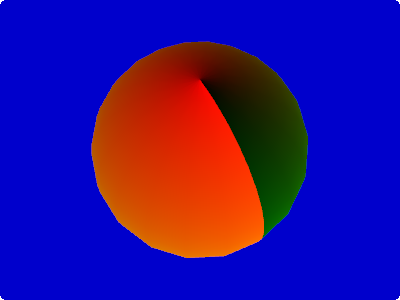You can see that the x coordinate of the texture is mapped to the red color of each pixel.
The y coordinate of the texture is mapped to the green color of each pixel.

Convert noise into colors

The next step is to use a noise function that we will then use to create the pseudo-random oceans and continents.

You can find an excellent noise function in the webgl-noise project.

Copy and paste the source code from the file noise2D.glsl into your shader.

#ifdef GL_ES
#define LOWP lowp
#define MED mediump
#define HIGH highp
precision mediump float;
#else
#define MED
#define LOWP
#define HIGH
#endif

varying MED vec2 v_texCoords0;

// INSERT HERE THE NOISE FUNCTIONS ...

float pnoise2(vec2 P, float period) {
return pnoise(P*period, vec2(period, period));
}

float earthNoise(vec2 P) {
vec2 r1 = vec2(0.70, 0.82); // random numbers

float noise = 0.0;
noise += pnoise2(P+r1, 9.0);

return noise;
}

void main() {
float noise = earthNoise(v_texCoords0);

gl_FragColor.rgb = noise;
}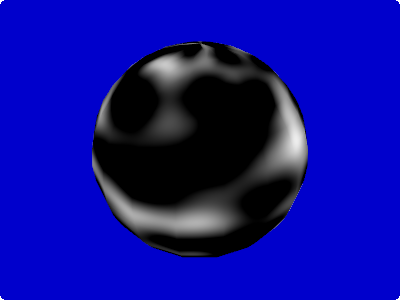Obviously the noise value 1.0 corresponds to white (= vec3(1.0, 1.0, 1.0)), while noise value 0.0 corresponds to black (= vec3(0.0, 0.0, 0.0)).
By now you might be wondering why the black areas are so large – is the noise function faulty?
Actually the noise function returns values in the range -1.0 to 1.0. But the conversion to RGB colors clamps all negative values to 0.0, hence the large black areas.

As an exercise to prove this (and as a tool to debug negative values in the future) let’s write a function that converts positive values (0.0 to 1.0) into green colors and negative values (-1.0 to 0.0) into red colors.

// lots of code omitted ...

vec3 toColor(float value) {
float r = clamp(-value, 0.0, 1.0);
float g = clamp(value, 0.0, 1.0);
float b = 0.0;
return vec3(r, g, b);
}

void main() {
float noise = earthNoise(v_texCoords0);

gl_FragColor.rgb = toColor(noise);
}

Hint: Try to avoid constructs using if because the GPU doesn’t like branching.
Instead of using if branching you should try to implement your functionality with the provided mathematical functions (clamp, mix, step, smoothstep, … ).
For a useful reference see: OpenGL ES Shading Language Built-In Functions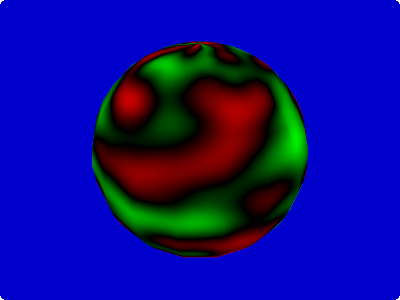Convert height into colors

We want to treat the result of the noise function as the height of the planet and map this height into the typical colors.
The easiest way to implement a function of an input value into a color is to use a one-dimensional texture.The x-axis of the texture corresponds to the height of the planet.

Until about 0.45 we paint all the heights the same deep blue of the ocean, then a few pixels of turquoise for the coastal waters, various green and yellows for the flora and deserts closer to the coast, then a large dark green block for the deep forest, finishing the whole with some grey mountains and a single white pixel for the snow at the top.

In the java code that defines the material we need now to specify this texture.

createTest(new UberShaderProvider("planet_step3"), new Material(new TextureAttribute(TextureAttribute.Diffuse, new Texture("data/textures/planet_height_color.png"))), Usage.Position | Usage.Normal | Usage.TextureCoordinates);
// lots of code omitted ...

void main() {
float noise = earthNoise(v_texCoords0);

vec3 color = texture2D(u_diffuseTexture, vec2(clamp(noise, 0.0, 1.0), 0.0));

gl_FragColor.rgb = color;
}

We do a lookup with texture2D() using the noise value as x-coordinate of the texture.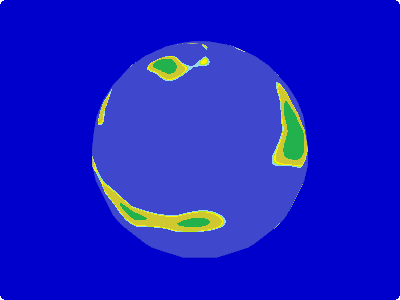Tweak the noise frequencies

Now it is time to make our continents a bit more convincing.
We want a couple of big continents with coastal areas that vary from smooth like the coasts of south-western Africa to fragmented like the fjords of Norway.

After some experiments I liked the following result:

float earthNoise(vec2 P) {
vec2 r1 = vec2(0.70, 0.82);
vec2 r2 = vec2(0.81, 0.12);
vec2 r3 = vec2(0.24, 0.96);
vec2 r4 = vec2(0.39, 0.48);
vec2 r5 = vec2(0.02, 0.25);
vec2 r6 = vec2(0.77, 0.91);
vec2 r7 = vec2(0.48, 0.05);
vec2 r8 = vec2(0.82, 0.48);

float noise = 0.0;
noise += clamp(pnoise2(P+r1, 3.0), 0.0, 0.45); // low-frequency noise clamped just slightly above ocean level - this produces the continental plates
noise += pnoise2(P+r2, 9) * 0.7; // medium frequency noise to produce the high mountain ranges (can be under and above water)
noise += pnoise2(P+r3, 14) * 0.2 + 0.1; // medium frequency noise for some hilly regious
noise += smoothstep(0.0, 0.1, pnoise2(P+r4, 8.0)) * pnoise2(P+r5, 50.0) * 0.3; // high frequency noise - but not in all areas
noise += smoothstep(0.0, 0.1, pnoise2(P+r6, 11.0)) * pnoise2(P+r7, 500.0) * 0.01;  // very high frequency noise - but not in all areas
noise += smoothstep(0.8, 1.0, noise) * pnoise2(P+r8, 350.0) * -0.3;  // very high frequency noise - only in the highest mountains

return noise;
}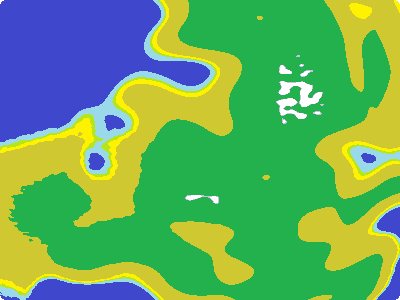The vectors r1 to r8 are random numbers so that not all our generated planets will look the same.
Later we can make then into uniforms and control them from the Java application.

If you want to understand how the different noise parts contribute to the total noise you can use the toColor() function to debug it visually.
Comment out all the other noise components and feed the final result into toColor().

noise += clamp(pnoise2(P+r1, 3.0), 0.0, 0.45); // low-frequency noise clamped just slightly above ocean level - this produces the continental plates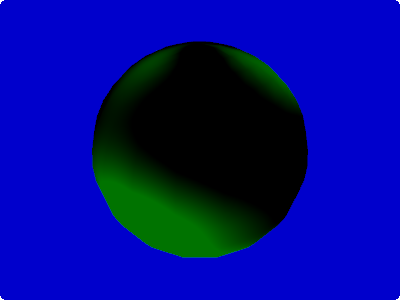noise += pnoise2(P+r2, 9) * 0.7; // medium frequency noise to produce the high mountain ranges (can be under and above water)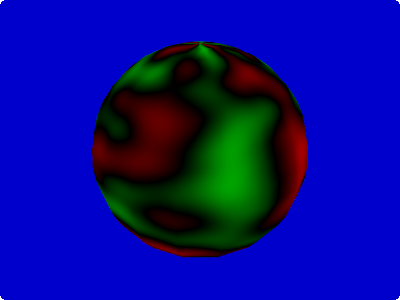noise += pnoise2(P+r3, 14) * 0.2 + 0.1; // medium frequency noise for some hilly regious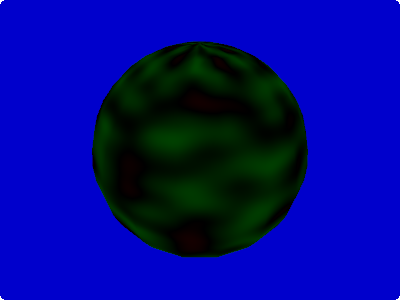noise += smoothstep(0.0, 0.1, pnoise2(P+r4, 8.0)) * pnoise2(P+r5, 50.0) * 0.3; // high frequency noise - but not in all areas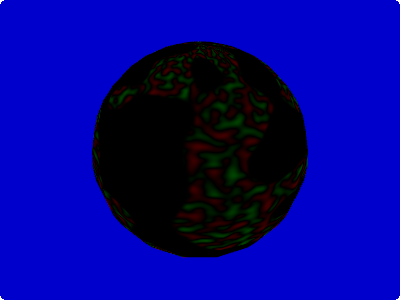The very high frequency with an amplitute of 0.01 is not visible with the color range of our toColor() function
and the last smoothstep() uses the calculated noise so that only the high mountain ranges receive the high frequency noise.
If you want to make these visible you need play around with the functions.

As last step lets have a look at the total output of the noise function using toColor().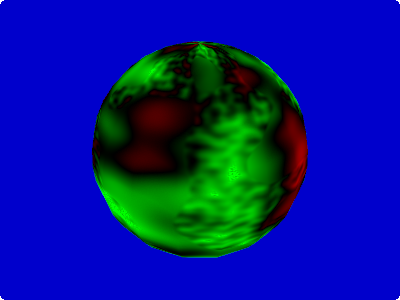Convert latitude and height into colors

Our planet already looks reasonable but if you look at a picture of earth you will immediately notice that the latitude also influences the color.
High in the north and south we have the polar caps and closer to the equator we see large desert areas.

To implement this we can use a 2 dimensional texture.

As before it encodes in the x-axis the height of the plant, on the y-axis corresponds to the distance to the equator.We see the desert and steppe close to the equator. In the medium latitudes forest becomes predominant before it is replaced by tundra and finally the ice cap at the pole.

Let’s do the two-dimensional lookup in this texture.

void main() {
float noise = earthNoise(v_texCoords0);

float distEquator = abs(v_texCoords0.t - 0.5) * 2.0;
vec3 color = texture2D(u_diffuseTexture, vec2(clamp(noise, 0.0, 1.0), distEquator));

gl_FragColor.rgb = color;
}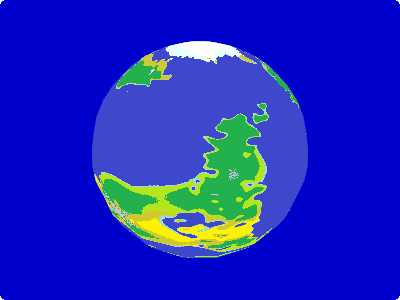By changing the calculation of the distance to equator we can change the overall climate of the planet.
Let’s have a look how it looks during an ice age.

void main() {
float distEquator = abs(v_texCoords0.t - 0.5) * 6.0;
}Actually we can make the whole planet look much nicer by running a gaussian blur over the texture, so that the colors of the different bio zones mix with each other.
Please note that the coast is not blurred into the water.Here some more screenshots using the blurred texture.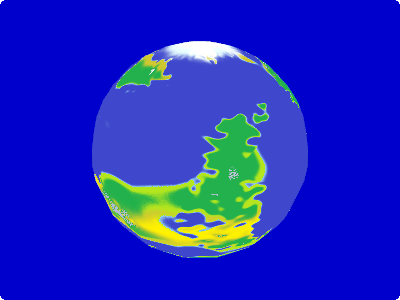Posted in Development, GLSL, Java, LibGDX | 1 Comment

Memory Impact of Java Collections

Sometimes it is important to know how much memory you need in order to store the data in a Java collection.

Here is a little overview of the memory overhead of the most important Java collections.

In some cases I also added my own implementations to see how they shape up.

All collections where filled with 10000 Integer elements and then the memory was measured by producing a heap dump and then analyzing the heap dump with MemoryAnalyzer.
Executed in:

Java(TM) SE Runtime Environment (build 1.6.0_23-b05)
Java HotSpot(TM) 64-Bit Server VM (build 19.0-b09, mixed mode)

on a

Intel(R) Core(TM) i7 CPU       M 620  2.67GHz (4 CPUs), ~2.7GHz

Set

The data stored in the sets is always the same: 10000 instances of Integer:

Class Name Objects Bytes
java.util.Integer 10,000 240,000
Total 10,000 240,000

Memory Footprint

java.util.HashSet

Class Name Objects Bytes
java.util.HashMap$Entry 10,000 480,000 java.util.HashMap$Entry[] 1 131,096
java.util.HashMap 1 64
java.util.HashSet 1 24
java.util.HashMap$KeySet 1 24 Total 10,004 611,208 java.util.TreeSet Class Name Objects Bytes java.util.TreeMap$Entry 10,000 640,000
java.util.TreeMap 1 80
java.util.TreeSet 1 24
Total 10,002 640,104

Collections.synchronizedSet(java.util.HashSet)

A synchronized HashSet by wrapping it with the Collections.synchronizedSet() method.

Class Name Objects Bytes
java.util.HashMap$Entry 10,000 480,000 java.util.HashMap$Entry[] 1 131,096
java.util.HashMap 1 64
java.util.Collections$SynchronizedSet 1 32 java.util.HashSet 1 24 Total 10,004 611,208 Collections.newSetFromMap(ConcurrentHashMap) Java does not provide a ConcurrentHashSet out of the box, but you can create an equivalent by wrapping a ConcurrentHashMap with Collections.newSetFromMap(). Class Name Objects Bytes java.util.concurrent.ConcurrentHashMap$HashEntry 10,000 480,000
java.util.concurrent.ConcurrentHashMap$HashEntry[] 16 131,456 java.util.concurrent.ConcurrentHashMap$Segment 16 768
java.util.concurrent.ConcurrentHashMap$NonfairSync 16 768 java.util.concurrent.ConcurrentHashMap$Segment[] 1 152
java.util.concurrent.ConcurrentHashMap 1 72
java.util.Collections$SetFromMap 1 32 java.util.concurrent.ConcurrentHashMap$KeySet 1 24
Total 10,052 613,272

ch.obermuhlner.collection.ArraySet

This is an experimental implementation of an array-based mutable Set that was designed to have minimal memory footprint.

Access with contains() is O(n).

Class Name Objects Bytes
java.lang.Object[] 1 80,024
ch.obermuhlner.collection.ArraySet 1 24
Total 2 80,048

ch.obermuhlner.collection.ImmutableArraySet

Similar to the ArraySet above but immutable. Designed for minimal memory footprint.

Access with contains() is O(n).

Class Name Objects Bytes
java.lang.Object[] 1 80,024
ch.obermuhlner.collection.ImmutableArraySet 1 24
Total 2 80,048

ch.obermuhlner.collection.ImmutableSortedArraySet

Another experimental implementation of an array-based immutable Set.
The array is sorted by hash code and contains() uses a binary search.

Access with contains() is O(log(n)).

The ImmutableSortedArraySet has the option to store the hash codes of all elements in a separate int[] which trading improved performance with additional memory footprint.

Class Name Objects Bytes
java.lang.Object[] 1 80,024
int[] 1 40,024
ch.obermuhlner.collection.ImmutableSortedArraySet 1 32
Total 3 120,080

Performance

The performance of the different Sets was measured by running contains() with an existing random key against a set of a specific size.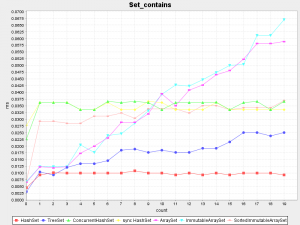Measuring with up to 20 elements shows that ArraySet and ImmutableArraySet really are linear. With less than 10 elements they are actually faster than the O(log(n)) ImmutableSortedArraySet.

In the next chart we see the performance with up to 1000 elements. The linear Sets are no longer shown because they out-scale everything else.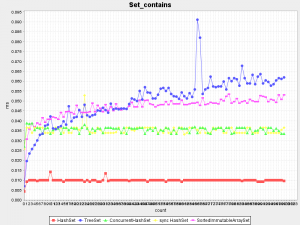Posted in Development, Java | 2 Comments

Benchmarking Scala

Microbenchmarking is controversial as the following links show:

Nevertheless I did write a little micro-benchmarking framework in Scala so I could experiment with the Scala language and libraries.

It allows to run little code snippets such as:

object LoopExample extends ImageReport {
def main(args: Array[String]) {
lineChart("Loops", 0 to 2, 0 to 100000 by 10000,
new FunBenchmarks[Int, Int] {
prepare {
count => count
}
run("for loop", "An empty for loop.") { count =>
for (i <- 0 to count) {
}
}
run("while loop", "A while loop that counts in a variable without returning a result.") { count =>
var i = 0
while (i < count) {
i += 1
}
}
})
}

This produced the image used in the last blog Scala for (i <- 0 to n) : nice but slow: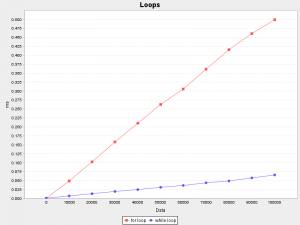The framework also allows to create an HTML report containing multiple benchmarks suites.

Let's have a look at some of the more interesting results.

Note: All micro-benchmarking results should always be interpreted with a very critical mindset. Many things can go wrong when measuring a single operation over and over again. It is very easy to screw up and become meaningless results.
If you want to analyze a particular benchmark in more details, follow the details link at the end of the suite chapter. It will show a detailed statistical analysis of this particular benchmark suite.

Loops

run("for loop", "An empty for loop.") { count =>
for (i <- 0 to count) {
}
}
run("for loop result", "A for loop that accumulates a result in a variable.") { count =>
var result = 0
for (i <- 0 to count) {
result += i
}
result
}
run("while loop", "A while loop that counts in a variable without returning a result.") { count =>
var i = 0
while (i < count) {
i += 1
}
}
run("while loop result", "A while loop that accumulates a result in a variable.") { count =>
var result = 0
var i = 0
while (i < count) {
result += i
i += 1
}
result
}
run("do while loop", "A do-while loop that counts in a variable without returning a result.") { count =>
var i = 0
do {
i += 1
} while (i <= count)
}
run("do while loop result", "A do-while loop that accumulates a result in a variable.") { count =>
var result = 0
var i = 0
do {
result += i
i += 1
} while (i <= count)
result
}

As already discussed in another blog entry, loops in Scala perform surprisingly different: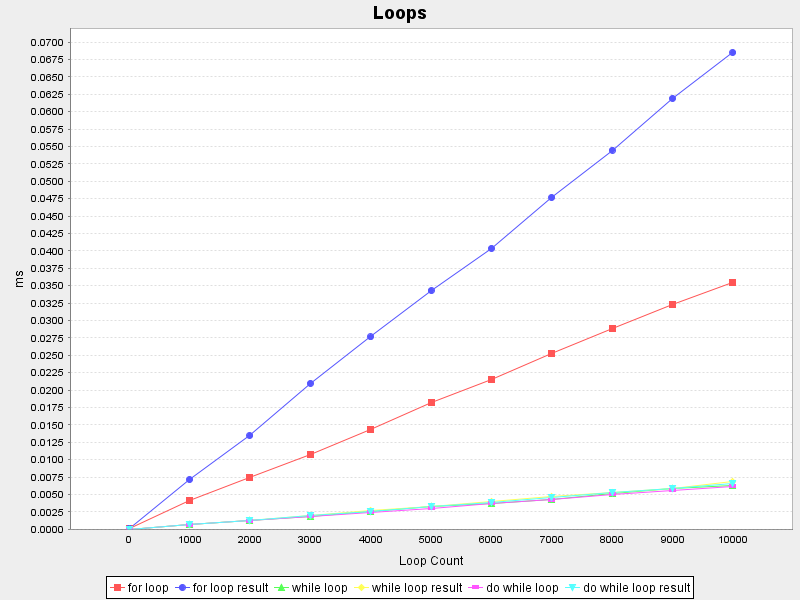The for (i <- 1 to count) loop is significantly slower than while (i < count).

Arithmetic

Not really a surprise, but BigDecimal arithmetic is very slow compared to double arithmetic (on both charts the red line is the reference benchmark that executes in the same time).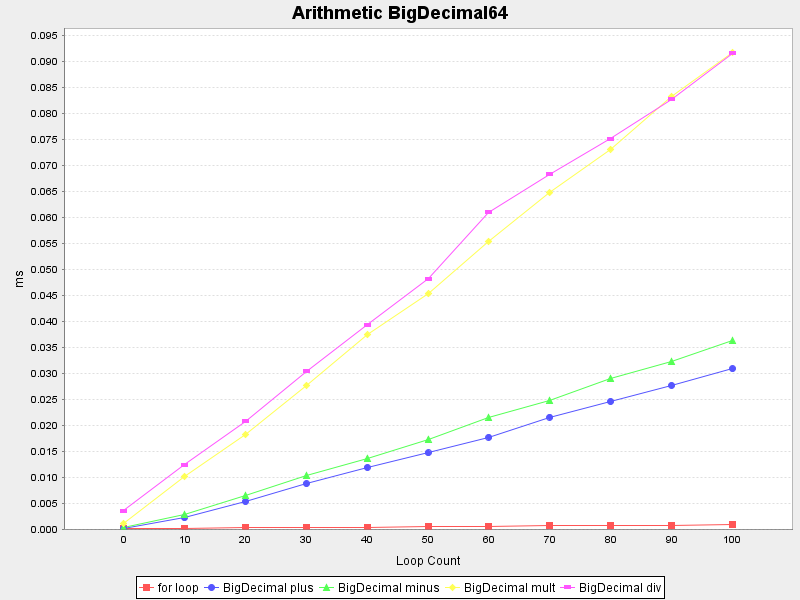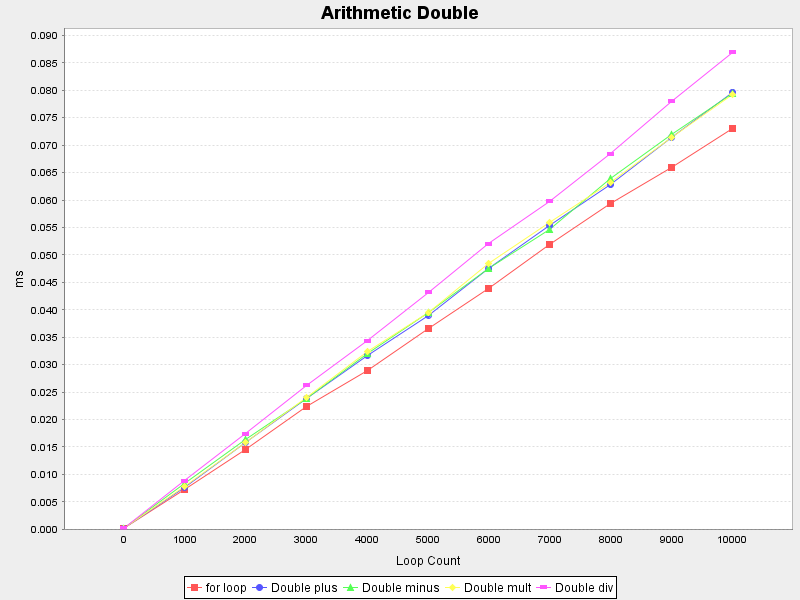Casts

I doubted the performance of the Scala way of casting a reference, so I compared it with the Java-like cast method:

var any: Any = "Hello"
var result: String = _

run("asInstanceOf", "Casts a value using asInstanceOf.") { count =>
for (i <- 0 to count) {
result = any.asInstanceOf[String]
}
}
run("match case", "Casts a value using pattern matching with the type.") { count =>
for (i <- 0 to count) {
result = any match {
case s: String => s
case _ => throw new IllegalArgumentException
}
}
}

Happily they perform practically the same: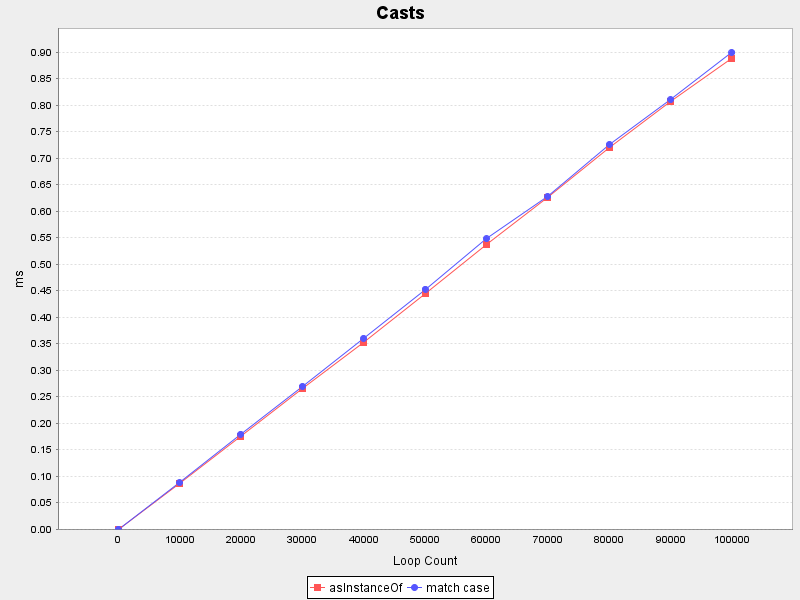Immutable Map

I am really fond of immutable maps, they are easy to reason use and perform very well.

run("contains true", "Checks that a map really contains a value.") { map =>
map.contains(0)
}
run("contains false", "Checks that a map really does not contain a value.") { map =>
map.contains(-999)
}
run("+", "Adds a new entry to a map.") { map =>
map + (-1 -> "X-1")
}
run("-", "Removes an existing entry from a map.") { map =>
map - 0
}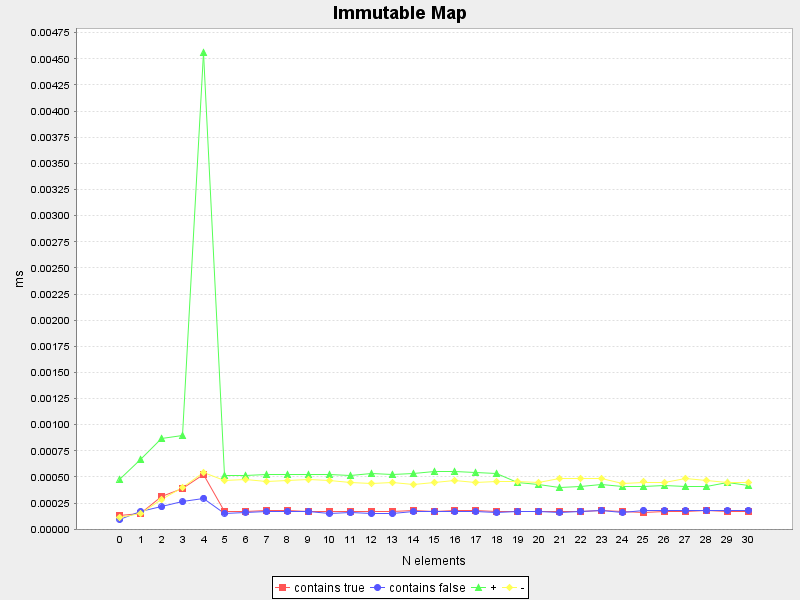The immutable maps with size 0 to 4 are special classes that store the key/value pairs directly in dedicated fields (testing sequentially) - therefore we see linear behaviour there.

The strong peak when adding another key/value pair to an immutable map with a size of 4 is probably because it switches to the normal scala.collection.immutable.HashMap (and creating 4 tuples) after testing all keys:

// Implementation detail of scala.collection.immutable.Map4
override def updated [B1 >: B] (key: A, value: B1): Map[A, B1] =
if (key == key1)      new Map4(key1, value, key2, value2, key3, value3, key4, value4)
else if (key == key2) new Map4(key1, value1, key2, value, key3, value3, key4, value4)
else if (key == key3) new Map4(key1, value1, key2, value2, key3, value, key4, value4)
else if (key == key4) new Map4(key1, value1, key2, value2, key3, value3, key4, value)
else new HashMap + ((key1, value1), (key2, value2), (key3, value3), (key4, value4), (key, value))
def + [B1 >: B](kv: (A, B1)): Map[A, B1] = updated(kv._1, kv._2)

HashMap

The standard Java java.util.HashMap is also interesting for Java programmers.

run("contains true", "Checks that a map really contains a value.") { map =>
map.containsKey(0)
}
run("contains false", "Checks that a map really does not contain a value.") { map =>
map.containsKey(-999)
}
run("size", "Calculates the size of a map.") { map =>
map.size()
}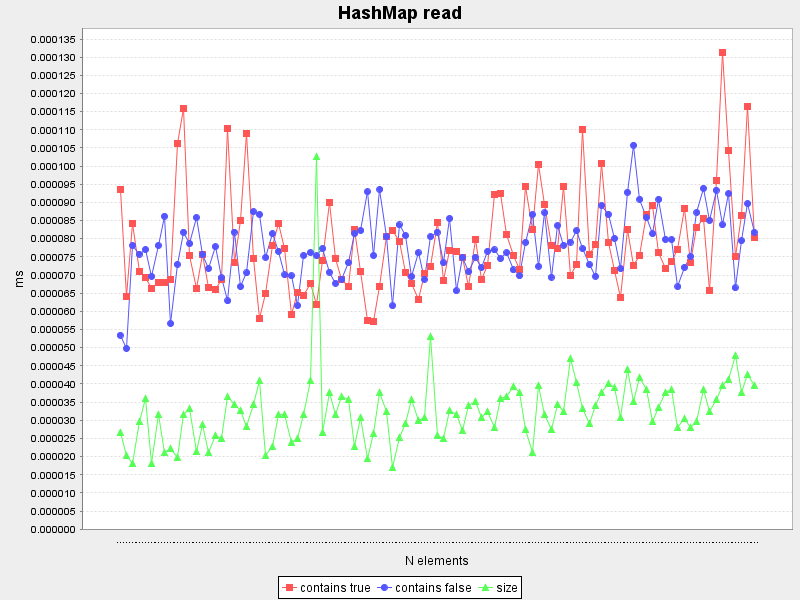At first glance containsKey and size() seem to be constant (as expected), but to be sure an additional benchmark with a larger n up to a 1000 was added: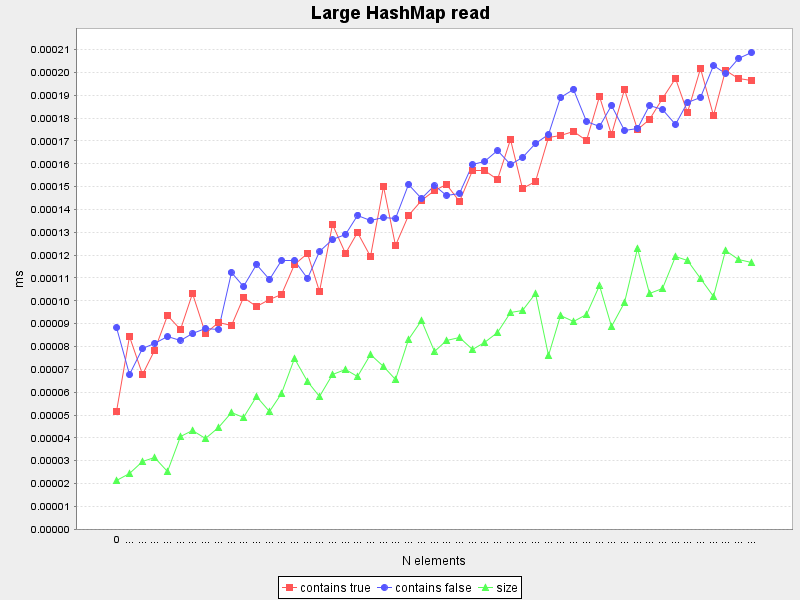Surprisingly all measured methods grow slowly with increasing size (contrary to the expected constant time behaviour) - this needs to be analyzed in detail.

A look at the details of this suite shows that actually all measured elapsed times are either 0.00000 ms or 0.00038 ms and the apparent increase with growing size is purely a statistical side effect. Obviously this benchmark is at the low end of measurement accuracy.

The measured write operations are:

run("put", "Adds a new entry to a map.") { map =>
map.put(-1, "X-1")
}
run("remove", "Removes an existing entry from a map.") { map =>
map.remove(0)
}
run("clear", "Removes all entries from a map.") { map =>
map.clear()
}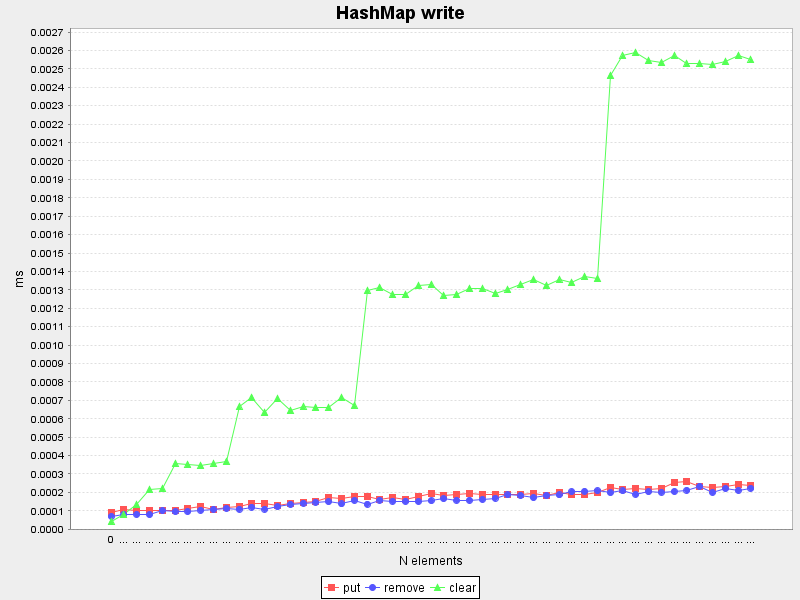put() and remove() are reasonably constant, albeit they grow very slowly with increasing size.

I was very surprised to see that the clear() method is not constant time. A quick look at the implementation shows that it is linear with the number of entries.

// Implementation of java.util.HashMap.clear()
public void clear() {
modCount++;
Entry[] tab = table;
for (int i = 0; i < tab.length; i++)
tab[i] = null;
size = 0;
}

ConcurrentHashMap

The behaviour of java.util.concurrent.ConcurrentHashMap is very similar to HashMap (slightly slower).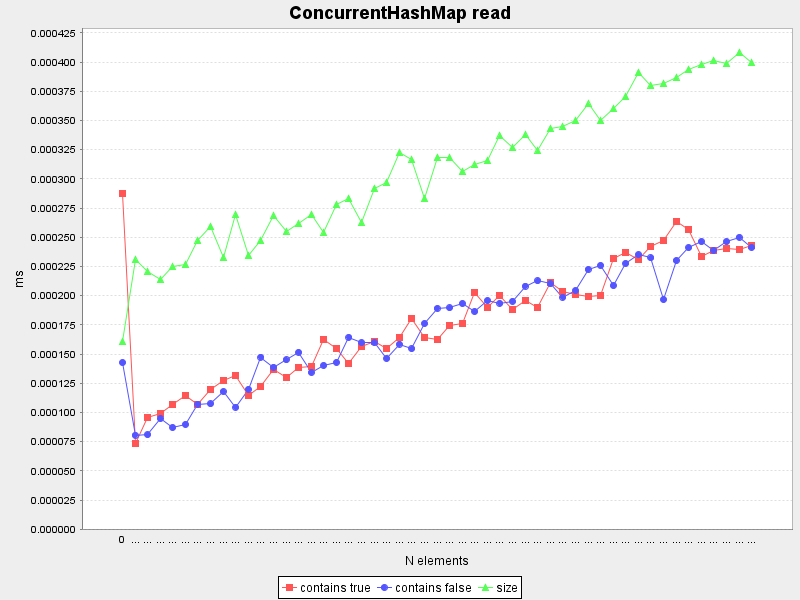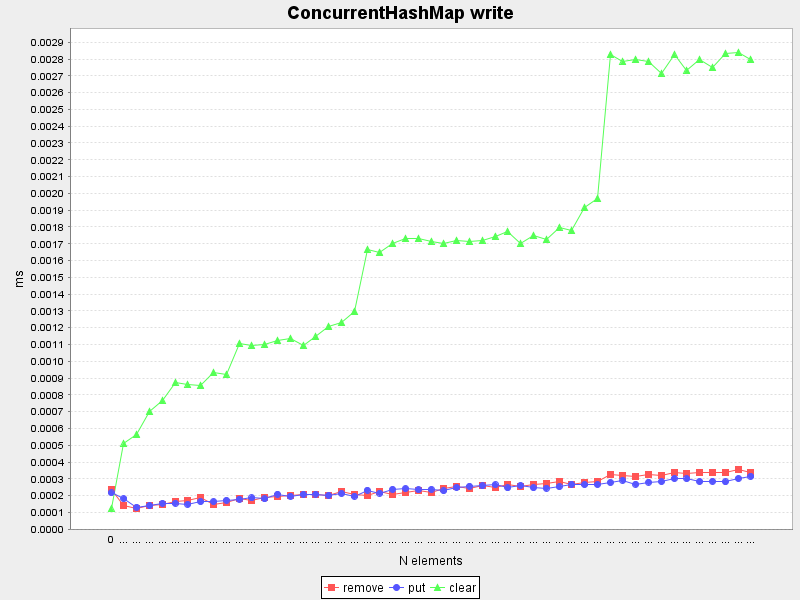Pattern Matching

Pattern matching is a very nice and powerful feature of Scala.

This benchmark tests only the simplest case of pattern matching and compares them with a comparable if-else cascade.

run("match 1", "Matches the 1st pattern with a literal integer value.") { seq =>
for (value <- seq) {
value match {
case 1 => "one"
case _ => "anything"
}
}
}
run("if 1", "Matches the 1st if in an if-else cascade with integer values.") { seq =>
for (value <- seq) {
if (value == 1) "one"
else "anything"
}
}
run("match 5", "Matches the 5th pattern with a literal integer value.") { seq =>
for (value <- seq) {
value match {
case 1 => "one"
case 2 => "two"
case 3 => "three"
case 4 => "four"
case 5 => "five"
case _ => "anything"
}
}
}
run("if 5", "Matches the 5th if in an if-else cascade with integer values.") { seq =>
for (value <- seq) {
if (value == 1) "one"
else if (value == 2) "two"
else if (value == 3) "three"
else if (value == 4) "four"
else if (value == 5) "five"
else "anything"
}
}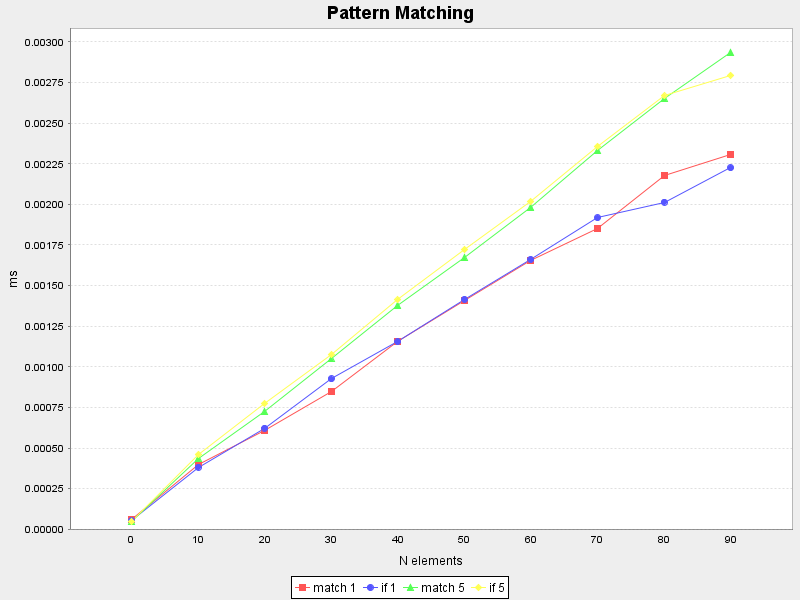As you see, simple pattern matching and the if-else cascade have comparable speed.